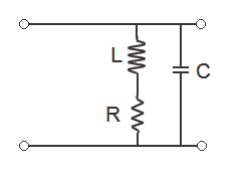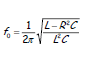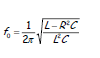RLC circuit and resonance frequency

• Engineering

Homework StatementFind an equation for the resonance frequency. Then find R L and C given that the resonance freq is 10 Hz.

Wrote below

The Attempt at a Solution

y(jw) = (1 / R + jwl) + jwC is the basic equation.. after simplifying and setting imaginary part to 0 and changing w to f I get
RF = 1/2pi * ((L-R^2C)/(L^2*C))^1/2

I'm pretty sure that part is correct.. how do I find the values for R L and C using this now?

rude man
Homework Helper
Gold Member
I don't believe R, L and C are uniquely derivable from just the resonant freq.

For example, 1/2π√(LC) = 10Hz with R = 0 is one solution. Pick any L or C, then the other is determined.

To include a finite R, adjust L or C slightly.

Dumb problem.

gneill
Mentor
It's a rather vague problem in its given form, but in a real life situation you might be required to design a circuit where one or more of the parts happen to be "given" because they happen to be in stock or otherwise the only ones available. For example, you might have a 10mH coil in hand, and being a real-life coil would have an inherent winding resistance. That would start you off with L and R as givens.

I don't believe R, L and C are uniquely derivable from just the resonant freq.

For example, 1/2π√(LC) = 10Hz with R = 0 is one solution. Pick any L or C, then the other is determined.

To include a finite R, adjust L or C slightly.

Dumb problem.

I get what your saying, if we assume that the resonance frequency is given with the following formula

f_o= sqrt(1/LC).

We are asked to find the values of L and C for a resonance frequency of 10 Hz. The solution is not unique as we are given two unknowns and there is one known. For instance 0.1H and 0.1F for the inductor and capacitor respectively satisfy the condition for 10Hz. The problem does not say whether R needs to be finite or not so I'm not quite sure..That's my expression for the resonance frequency.. So do I just pick a value for C, say 0.1F and then solve for L, and say R is 0?

And to include a finite R, how do I adjust L and C. For example in the basic expression above with 0.1H and 0.1F satisfying the condition for 10Hz, how do you determine what R is?

rude man
Homework Helper
Gold Member
I get what your saying, if we assume that the resonance frequency is given with the following formula

f_o= sqrt(1/LC).

We are asked to find the values of L and C for a resonance frequency of 10 Hz. The solution is not unique as we are given two unknowns and there is one known. For instance 0.1H and 0.1F for the inductor and capacitor respectively satisfy the condition for 10Hz. The problem does not say whether R needs to be finite or not so I'm not quite sure..That's my expression for the resonance frequency.. So do I just pick a value for C, say 0.1F and then solve for L, and say R is 0?
You can certainly do that.

And to include a finite R, how do I adjust L and C. For example in the basic expression above with 0.1H and 0.1F satisfying the condition for 10Hz, how do you determine what R is?

Pick any R and C and solve for L. Or, more realistically, as gneill suggests, pick his L, add a few R and compute C using your formula again.

Pick any R and C and solve for L. Or, more realistically, as gneill suggests, pick his L, add a few R and compute C using your formula again.

Alright thanks for the help. Choosing R = 1 ohm and L = 0.1H I get 0.002F for C. The professor just sent out an email a bit ago saying that the solution is not unique as well so that clarifies the problem.